Latest Teaching jobs   »   CTET 2012 Maths Pedagogy Questions: Free...

# CTET 2012 Maths Pedagogy Questions: Free PDF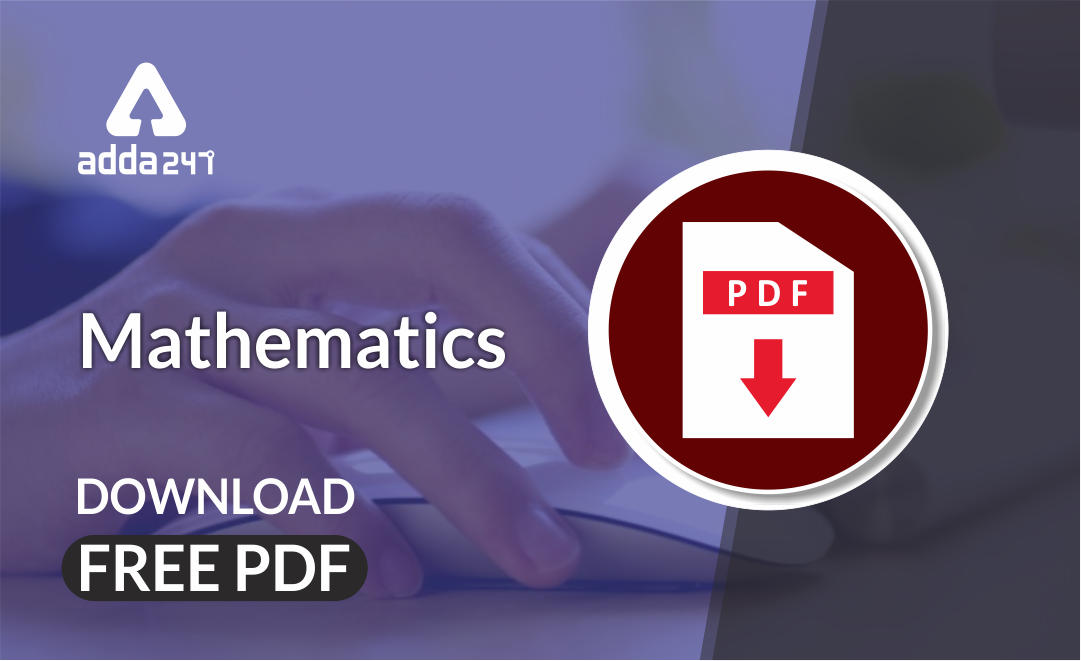Mathematics is an equally important section for CTET, MPTET, KVS & DSSSB Exams and has even more abundant importance in some other exams conducted by central or state govt.

To let you make the most of Mathematics section, we are providing important previous year Maths Pedagogy Questions.  We wish you all the best of luck to come over the fear of the Mathematics section.

Mathematics Study Notes For All Teaching Exams

## CTET 2012(Paper 2) Maths Pedagogy Questions

Q1. Salman solves – 3 – 4 = +7. The error is committed as

(a) Salman has not understood the concept of multiplication of integers

(b) Salman is careless

(c) Salman is not clear about the concept of addition of integers

(d) Salman needs to practice solving problems of similar type

Q2. Problem solving method is the

(a) cultivation of mathematical ingenuity, creativity and heuristic thinking by making students open – minded

(b) gradual and systematic guiding through the hierarchy of mathematical notions, ideas and techniques

(c) teaching of mathematical results, definitions and concepts by repetition and memorization

(d) teaching the development of mathematics within a historical, social and cultural context

Q3. While solving a problem based on ‘Pythagoras theorem’, a teacher draws the following triangle ABC. Rajan argued that the triangle ABC is not drawn correctly. The only way to draw it is Rajan has the misconception as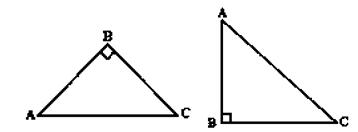(a) he lacks in analytical ability

(b) he is weak in geometrical concepts

(c) his teacher must have always drawn the triangle in this particular way

(d) he has dysgraphia

Q4. In order to help the students with difficulties in remembering the geometrical terms and their meaning, a teacher

(a) encourage group discussions

(b) stress on rote memorization of all terms and definitions

(c) use lots of activities like preparing or solving crossword puzzles, jig – saw puzzles, etc.

(d) test students on definition of geometrical terms

Q5. Mr. Manish used lots of manipulative, Maths Lab activities and ICT activities to clarify the concept of ‘Symmetry’. Mr. Manish wants to

(a) cater to kinesthetic learners only

(b) cater to students of all learning styles

(c) be popular amongst his students

(d) pass time so that he can avoid teaching of next topic as it is not of his interest

Q6. A student observed the following examples:

(10)² = (5 + 5)² = 5² +2(5)(5) + (5)² = 100

= (6 + 4)² = 6² +2(6)(4) + (4)² = 100

= (8 + 2)² = 8² + 2(8)(2) + (2)² = 100

= (1 + 9)² = 1² +2(1)(9) + (9)² = 100

The above method of drawing conclusions is

(a) Activity

(b) Deductive

(c) Inductive

(d) Analytical

Q7. Summative Assessment of the unit ‘Mensuration’ can be done through

(a) ICT activity
(b) Project work
(c) Maths Lab activity
(d) Paper – Pencil test

Q8. Ankur got zero marks in a word problem on linear equations in an assessment. The teacher knows that he can solve linear equations correctly. The teacher ought to remark in his report

(a) Ankur lacks concentration and hence has examination phobia

(b) Ankur lacks concentration and hence has examination phobia

(c) Ankur has not understood the concept of linear equations completely

(d) Ankur has a problem in comprehending the language of the question, though he can solve the equations

Q9. While teaching ratio and proportions, Ms. Rana demonstrated some computer operations on the screen ‘copy and paste’ and ‘copy and enlarge or copy and reduce’. This activity may be

(a) fun activity to pass time

(b) pre – content activity to introduce ratio

(c) post – content activity

(d) Ameena would be learning by doing and Appu may not be able to see the pattern at all

Q10. A suitable approach to introduce Coordinate Geometry in Class IX is through the use of

(a) Solving problems

(b) Lecture method

(c) Role play

(d) Demonstration using technology integration

Q11. “How does a square form a parallelogram? Explain.” The students are asked to write the answer to above question. The objective of the teacher is

(a) improving the writing skills of the students

(b) giving an opportunity to students to think and reflect

(c) making the classroom more interactive

(d) exposing the students to open – ended questions

Q12. A lesson plan on unit of Mensuration includes one of the instructional objectives as follows: “Learners will be able to understand the application of Mathematics.” This instructional objective is

(a) inappropriate, as the objective is vague and ill – defamed

(b) appropriate, as all units of Mathematics aim at this only

(c) appropriate, as unit on ‘Mensuration’ has lots of application in day – to – day life

(d) appropriate, as one must be able to apply the knowledge gained

Q13. In Class VII, a teacher taught the properties of all types of quadrilateral’. In the class test that followed after the unit, the teacher asked the problems on construction of quadrilateral. No one in the class was able to perform in the test. The reason may be

(a) the students were not serious about the class – test and had not prepared well

(b) all students of the class are dull

(c) there is a gap between instructions given in class and the assessment conducted

(d) the teacher is not able to create the interest of the students in this unit

Q14. Present NCERT text – books on Mathematics are written keeping in mind the recommendations of

(a) Syllabus prescribed by State Board in 2006

(b) National Curriculum Framework 2005

(c) National Policy on Education 1986

(d) Syllabus prescribed by CBSE in 2006

Q15. Read the following question :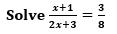Which cognitive skill of Bloom’s Taxonomy is emphasized in this question ?

(a) Analysis

(b) knowledge

(c) Comprehension

(d) Application

Q16. “The organisers of an essay competition decide that a winner gets a prize of 100 and a participant who does not win gets a prize of 25. The total prize money distributed is 3,000. Find the number of winners, if the total number of participants is 63.”

Apart from checking the mathematical concept, what values can a teacher inculcate through this question amongst students, during the discussions ?

(a) Writing of thoughts on social issues can be developed as a regular habit and the opportunities to make your thoughts public can be availed through participation in essay competition

(b) Winning is more important than participation

(c) Participation is more important

(d) Competency of writing a good essay is important

Q17. A teacher asked his/her students to complete the following concept map : With this activity in class, the teacher is using concept map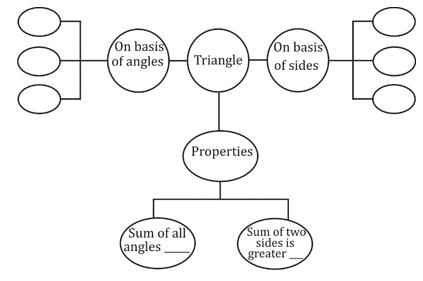(a) to enhance spatial ability of the learner so that she/he can see the complete unit as a whole

(b) to catch the attention of each student

(c) to provide different kinds of learning material

(d) to conduct formative assessment and to get insight on how much students have learnt

Q18. Identify the open – ended problem.

(a) Find the cube – root of 512 using prime factorisation method.

(b) Find the cube of 10

(c) Write any 5 numbers whose cube is more than 64.

(d) Parikshit makes a cuboid of dimension 5 cm, 2 cm, 5 cm. How many such cuboids are required to form a cube?

Q19. Portfolio of Mathematics students can have record of all class tests, unit tests conducted during the formative and summative assessment

(a) record of his photographs, drawing and art work

(b) record of classwork and homework notebooks

(c) record of assignments, worksheets, maths lab activity record, write – up of any mathematics

(d) model, mathematical posters and cards, any new type of problem attempted or

Q20. It is observed that to a problem like ‘Show that the sum of any two odd numbers is an even number’, most of the students replied by quoting one example, say, 5 + 7 = 12. Students answered this question inappropriately as

(a) students have attained the formal operational stage as proposed by Piaget

(b) students firmly believe that if a Statement is true for one set of numbers, it is always true and hence have developed strong strategy for making generalisations

(c) students have not learnt the logical proof for the statement in the class

(d) students have not understood that in Mathematics any’ is used to indicate generalisations and hence are not able to answer correctly

Mathematics Quiz For CTET Exam : Attempt Daily Quizzes

Solutions

S1. Ans.(c)

Sol. Salman is not clear about the concept of addition of integers.

S2. Ans.(b)

Sol. In mathematics, problem – solving method is the gradual and systematic guiding through the hierarchy of mathematical notions, ideas and techniques.

S3. Ans.(a)

Sol. Rajan argued with his teacher regarding the right angled triangle ABC not drawn correctly. So, Rajan lacks his analytical ability because we can draw right angled triangle taking any one of the angles as right angle.

S4. Ans.(c)

Sol. A teacher must use lots of activities like preparing or solving crossword puzzles, jig – saw puzzles etc. in order to help the students with difficulties in remembering the geometrical terms and their meaning

S5. Ans.(b)

Sol. To clarify the concept of ‘symmetry using manipulative, maths lab activities and ICT activities are very important methods to learn the concept of ‘Symmetry’ in practical manner. So, Mr. Manish wants cater to students of all learning styles.

S6. Ans.(c)

Sol. In inductive method the teacher first presents or takes the example from the students then comes on theory or concept. So, the method given in question is inductive method.

S7. Ans.(d)

Sol. Summative assessment of the unit ‘Mensuration cannot be possible without paper – pencil test.

S8. Ans.(d)

Sol. The questions related to linear equations are problem based. The word problem questions of linear equations cannot be solved without understanding the language of such questions Ankur got zero marks in a word problem of linear equations because he has a problem in understanding the language of the question, though he has ability to solve the linear equations.

S9. Ans.(b)

Sol. formative assessment activity

S10. Ans.(d)

Sol. Demonstration using technology integration

S11. Ans.(b)

Sol. Giving an opportunity to students to think and reflect

S12. Ans.(c)

Sol. This instructional object is appropriate as this is being done in our life in many situations. For example, Length of cloth required for stitching, the area of a wall that is being painted, perimeter of a circular garden to be fenced, capacity of water needed to fill the tank. For this kind of activities, we are doing measurements for further needs.

S13. Ans.(c)

Sol. There is a gap between instructions given in class and the assessment conducted

S14. Ans.(b)

Sol. National Curriculum Framework 2005

S15. Ans.(a)

Sol. There are six levels in the taxonomy, moving through the lowest order processes to the highest:

Knowledge : Exhibit memory of previously learned materials by recalling facts, terms, basic concepts and answers.

Questions like: What are the health benefits of eating apples

Comprehension : Demonstrate understanding of facts and ideas by organizing, comparing, translating, interpreting, giving descriptions, and stating the main ideas.

Questions like: Compare the health benefits of eating apples vs. oranges.

Application : Using new knowledge. Solve problems in new situations by applying acquired knowledge, facts, techniques and rules in a different way.

Questions like: Which kinds of apples are best for baking a pie, and why?

Analysis: Examine and break information into parts by identifying motives or causes. Make inferences and find evidence to support generalizations.

Questions like: List four ways of serving foods made with apples and explain which ones have the highest health benefits. Provide references to support your statements

Synthesis : Compile information together in a different way by combining elements in a new pattern or proposing alternative solutions.

Questions like: Convert an “unhealthy” recipe for apple pie to a “healthy” recipe by replacing your choice of ingredients. Explain the health benefits of using the ingredients you chose vs. the original ones.

Evaluation : Present and defend opinions by making judgments about information, validity of ideas or quality of work based on a set of criteria

Questions like: Do you feel that serving apple pie for an after school snack for children is healthy?

S16. Ans.(c)

Sol. Participation is more important

S17. Ans.(a)

Sol. Teacher is using concept map to enhance spatial ability to perceive or solve problems associated with relationships between objects or figures, including position, direction, size, form, and distance.

S18. Ans.(c)

Sol. Open – ended refers to a question or problem which has more than one correct answer and more than one strategy to obtain this answer. Therefore, write any 5 numbers whose cube is more than 64 is an example of open ended type questions.

S19. Ans.(d)

Sol. Portfolio of mathematics can a student Record of assignments, worksheets, maths lab activity record, write – up of any mathematics model, mathematical posters and cards, any new type of problem attempted or generalizations made etc.

S20. Ans.(c)

Sol. Students has not learnt the logical proof for the statement in the class.

•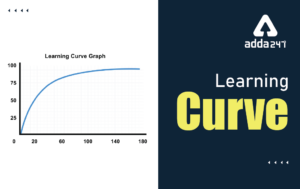Learning Curve - What is Learning Curve?...
•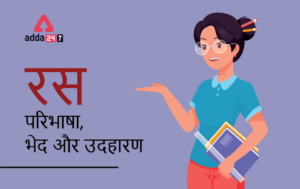रस - परिभाषा, भे�...
•पठन कौशल- परिभा�...
•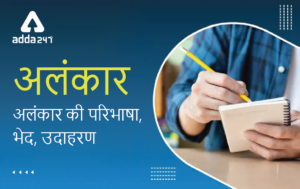अलंकार - परिभाष�...
•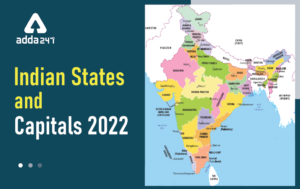Indian States and Capitals 2022: 28 Stat...
•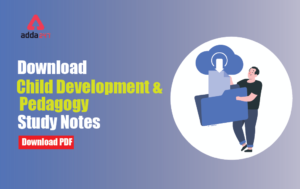New Education Policy(NEP) PDF - नई �...